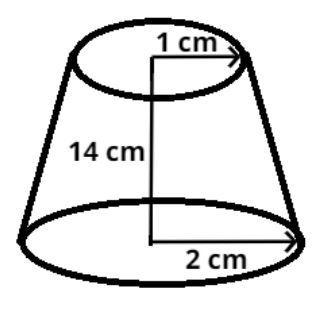Courses
Courses for Kids
Free study material
Free LIVE classes
MoreLIVE
Join Vedantu’s FREE Mastercalss

# A drinking glass is in the shape of a frustum of a cone of height $14cm$. The diameters of its two circular ends are $4cm$ and $2cm$. Find the capacity of the glass.Verified
366k+ views
Hint: - Use $\left[ {\pi = \dfrac{{22}}{7}} \right]$

The pictorial representation of the above problem is shown below.The height$\left( h \right)$ of the cone is $14cm$.
The diameters of its two circular ends are $4cm$ and $2cm$ respectively.
Radius $\left( {{r_1}} \right)$ of the lower circular part is$= \dfrac{{{\text{Diameter}}}}{2} = \dfrac{4}{2} = 2cm$.
Radius $\left( {{r_2}} \right)$of the upper circular part is$= \dfrac{{{\text{Diameter}}}}{2} = \dfrac{2}{2} = 1cm$
Volume of glass which is in the shape of frustum of cone$= \dfrac{1}{3}\pi h\left( {{r_1}^2 + {r_2}^2 + {r_1}{r_2}} \right)$
$= \dfrac{1}{3} \times \dfrac{{22}}{7} \times 14\left( {{2^2} + {1^2} + 2 \times 1} \right) \\ = \dfrac{{22 \times 14}}{3} = \dfrac{{308}}{3}c{m^3} \\$
So, this is the required volume of the glass.

Note: - In such types of problems the key concept is that always remember the formula of volume of frustum of cone which is stated above, then simplify we will get the required volume of the glass.

Last updated date: 28th Sep 2023
Total views: 366k
Views today: 9.66k